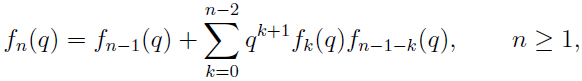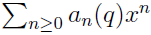# On a recurrence related to 321–avoiding permutations

Toufik Mansour and Mark Shattuck
Notes on Number Theory and Discrete Mathematics, ISSN 1310-5132
Volume 20, 2014, Number 2, Pages 74—78

## Details

### Authors and affiliations

Toufik MansourDepartment of Mathematics, University of Haifa
31905 Haifa, Israel

Mark ShattuckDepartment of Mathematics, University of Haifa
31905 Haifa, Israel

### Abstract

Dokos et al. recently conjectured that the distribution polynomial fn(q) on the set of permutations of size n avoiding the pattern 321 for the number of inversions is given by:with f0(q) = 1, which was later proven in the affirmative, see . In this note, we provide a new proof of this conjecture, based on the scanning-elements algorithm described in , and present an identity obtained by equating two explicit formulas for the generating function.

### Keywords

• Avoidance
• Inversion number
• q-analogue
• Continued fractions
• Permutations

• 11B37
• 11B65
• 05A15

### References

1. Cheng, S.-E., S. Elizalde, A. Kasraoui, B. E. Sagan. Inversion and major index polynomials, Preprint, http://arxiv.org/pdf/1112.6014.pdf.
2. Dokos, T., T. Dwyer, B. P. Johnson, B. E. Sagan, K. Selsor. Permutation patterns and statistics, Discrete Math., Vol. 312, 2012, 2760–2775.
3. Firro, G., T. Mansour. Three-letter-pattern-avoiding permutations and functional equations, Electron. J. Combin., Vol. 13, 2006, #R51.
4. Fürlinger, J., J. Hofbauer, q-Catalan numbers, J. Combin. Theory Ser. A, Vol. 40, 1985, No. 2, 248–264.

## Cite this paper

Mansour, T. & Shattuck, M. (2014). On a recurrence related to 321-avoiding permutations. Notes on Number Theory and Discrete Mathematics, 20(2), 74-78.